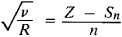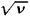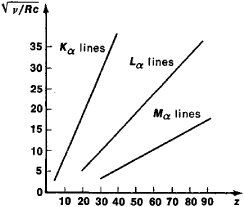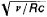# Moseley's Law

Also found in: Wikipedia.

## Moseley's law

[′mōz·lēz ‚lȯ]
(spectroscopy)
The law that the square-root of the frequency of an x-ray spectral line belonging to a particular series is proportional to the difference between the atomic number and a constant which depends only on the series.

## Moseley’s Law

a law that relates the frequency of the spectral lines of the characteristic X-radiation of a chemical element to its atomic number. This law was experimentally established by H. Moseley in 1913. According to Moseley’s law, the square root of the frequency v of a spectral line of the characteristic radiation of an element is a linear function of its atomic number Z:where R is the Rydberg constant, Sn is the screening constant, and n is the principal quantum number. On a Moseley plot (see Figure 1), the dependence ofon Z is a series of lines (such as the Kα lines, Lα lines, and Mα lines, which correspond to the values n = 1,2, and 3).

Moseley’s law was incontrovertible proof of the correctness of the arrangement of the elements in D. I. Mendeleev’s periodic system of the elements and the law helped to clarify the physical significance of Z.

According to Moseley’s law, the characteristic X-ray spectra do not display the periodic regularities that are inherent in optical spectra. This indicates that the inner electron shells of the atoms of all elements, which are manifested in the characteristic X-ray spectra, have an analogous structure.

Subsequent experiments revealed some deviations from a linearFigure 1.

Note to Figure 1: Moseley plot for the Kα lines, Lα lines, and Mα lines of characteristic X-radiation. The atomic number of the element Z is plotted along the axis of abscissas and the quantity(where c is the velocity of light) is plotted along the axis of ordinates.

dependence for the transition groups of elements—the deviations being due to the change in the order in which the outer electron shells are filled—and also for heavy atoms, in which the deviations result from relativistic effects (and may provisionally be explained by the fact that the velocities of the inner electrons are comparable with the velocity of light).

The position of the spectral lines on a Moseley plot may vary somewhat, depending on a number of factors, such as the number of nucleons in the nucleus (isotope shift) and the state of the outer electron shells (chemical shift). The study of these shifts makes it possible to obtain detailed information about the atom.

A. V. KOLPAKOV

Site: Follow: Share:
Open / Close